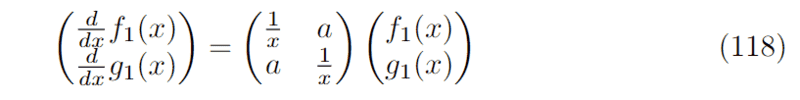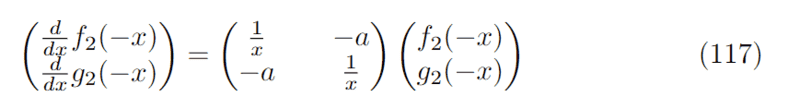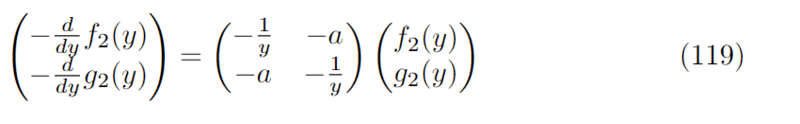# Quick Change of Variables Question

• I
• thatboi
I don't know what that means. Note that ##x## and ##y## are dummy variables. They have no intrinsic meaning, other than as arguments for your functions.For example, if we have ##f(-x) = -x##, then that is equivalent to ##f(x) = x##. Draw the graphs if you need to.

#### thatboi

Hey all,
I am currently struggling with a change of variables step in my calculations.
Suppose the solutions ##f_{1}(x)## and ##f_{2}(x)## of the following system of differential equations is known:Now the system I wish to solve is:Upon first glance, it seems that the association ##f_{2}(-x) \leftrightarrow f_{1}(x)## and ##g_{2}(-x) \leftrightarrow g_{1}(x)## will do the trick and we would be done.
However, I tried making the change of variables ##y=-x## in equation (117) and got the following:which is now the same form as equation (118).
Suddenly, it seems like the solution is now ##f_{2}(y) = f_{2}(-x) \leftrightarrow f_{1}(x)## and ##g_{2}(y) = g_{2}(-x) \leftrightarrow g_{1}(x)##
and I am left with a contradiction. Could someone point out to me where the mistake is? I feel like it is something simple that I am not seeing.
Thanks!

thatboi said:
Hey all,
I am currently struggling with a change of variables step in my calculations.
Suppose the solutions ##f_{1}(x)## and ##f_{2}(x)## of the following system of differential equations is known:
View attachment 313838
Now the system I wish to solve is:
View attachment 313839
Upon first glance, it seems that the association ##f_{2}(-x) \leftrightarrow f_{1}(x)## and ##g_{2}(-x) \leftrightarrow g_{1}(x)## will do the trick and we would be done.
However, I tried making the change of variables ##y=-x## in equation (117) and got the following:
View attachment 313840
which is now the same form as equation (118).
Suddenly, it seems like the solution is now ##f_{2}(y) = f_{2}(-x) \leftrightarrow f_{1}(x)## and ##g_{2}(y) = g_{2}(-x) \leftrightarrow g_{1}(x)##
and I am left with a contradiction. Could someone point out to me where the mistake is? I feel like it is something simple that I am not seeing.
Thanks!
Sorry there was a typo here, the second sentence above should have said "Suppose the solutions ##f_{1}(x)## and ##g_{1}(x)## off the following system of differential equations is known..."

thatboi said:
Hey all,
I am currently struggling with a change of variables step in my calculations.
Suppose the solutions ##f_{1}(x)## and ##f_{2}(x)## of the following system of differential equations is known:
View attachment 313838
Now the system I wish to solve is:
View attachment 313839
Upon first glance, it seems that the association ##f_{2}(-x) \leftrightarrow f_{1}(x)## and ##g_{2}(-x) \leftrightarrow g_{1}(x)## will do the trick and we would be done.
However, I tried making the change of variables ##y=-x## in equation (117) and got the following:
View attachment 313840
which is now the same form as equation (118).
Suddenly, it seems like the solution is now ##f_{2}(y) = f_{2}(-x) \leftrightarrow f_{1}(x)## and ##g_{2}(y) = g_{2}(-x) \leftrightarrow g_{1}(x)##
and I am left with a contradiction. Could someone point out to me where the mistake is? I feel like it is something simple that I am not seeing.
Thanks!

These functions depend on the parameter $a$; suppressing that dependence may lead you astray.

If we define $f_3(x;a) = f_2(-x;a)$ then (117) becomes $$\begin{pmatrix} \frac{d}{dx}f_3(x;a) \\\frac{d}{dx}g_3(x;a)\end{pmatrix} = \begin{pmatrix} 1/x & -a \\ -a & 1/x\end{pmatrix} \begin{pmatrix} f_3(x;a) \\ g_3(x;a) \end{pmatrix}.$$ It is then obvious that this is the same as (118) with $a$ replaced by $-a$. So $$f_2(-x;a) = f_3(x;a) = f_1(x;-a).$$

PeroK said:
Sorry there was another typo in my post, it should say "Upon first glance, it seems the association ##f_{2}(-x) \leftrightarrow f_{1}(x)## and ##g_{2}(-x) \leftrightarrow -g_{1}(x)## will do the trick..."
So there is a missing negative sign for ##g_{2}(-x)## between the 2 associations.

Last edited:
thatboi said:
Sorry there was another typo in my post, it should say "Upon first glance, it seems the association ##f_{2}(-x) \leftrightarrow f_{1}(x)## and ##g_{2}(-x) \leftrightarrow -g_{1}(-x)## will do the trick..."
So there is a missing negative sign for ##g_{2}(-x)## between the 2 associations.
I'm not sure what you mean by these "associations". You're looking an equation, such as ##f_2(x) = f_1(x)## or ##f_2(x) = -f_1(x)## or something like that.

PeroK said:
I'm not sure what you mean by these "associations". You're looking an equation, such as ##f_2(x) = f_1(x)## or ##f_2(x) = -f_1(x)## or something like that.
Yes, by association I mean like ##f_{2}(-x)## would be the same as ##f_{1}(x)## in form but just with ##x## swapped for ##-x## and ##g_{2}(-x) = -g_{1}(x)##

thatboi said:
Yes, by association I mean like ##f_{2}(-x)## would be the same as ##f_{1}(x)## in form but just with ##x## swapped for ##-x## and ##g_{2}(-x) = -g_{1}(x)##
I don't know what that means. Note that ##x## and ##y## are dummy variables. They have no intrinsic meaning, other than as arguments for your functions.

For example, if we have ##f(-x) = -x##, then that is equivalent to ##f(x) = x##. Draw the graphs if you need to. It's the same function.

PeroK said:
For example, if we have ##f(-x) = -x##, then that is equivalent to ##f(x) = x##. Draw the graphs if you need to. It's the same function.
I don't quite understand. So the situation was, if I knew the solutions ##f_{1}(x)## and ##g_{1}(x)## to the system in equation (118) and now I have a separate system equation (117) that I wish to find the solutions to. Then I can notice that because (118) and (117) have the same forms, the solution to equation (117) is just ##f_{2}(-x) = f_{1}(x)## and ##g_{2}(-x) = -g_{1}(x)##, that is, I can write the solution to (117) in terms of solutions to (118). But once I made a change of variables to change equation (117) to (119) and I try to write the solution to equation (119) in terms of the solution to equation (118), I end up with a different set of solutions.

thatboi said:
I don't quite understand. So the situation was, if I knew the solutions ##f_{1}(x)## and ##g_{1}(x)## to the system in equation (118) and now I have a separate system equation (117) that I wish to find the solutions to. Then I can notice that because (118) and (117) have the same forms, the solution to equation (117) is just ##f_{2}(-x) = f_{1}(x)## and ##g_{2}(-x) = -g_{1}(x)##, that is, I can write the solution to (117) in terms of solutions to (118). But once I made a change of variables to change equation (117) to (119) and I try to write the solution to equation (119) in terms of the solution to equation (118), I end up with a different set of solutions.
You have to do a correct change of variable. That means changing the derivative approriately. For example,$$\frac d {dx}f(-x) = -x$$is not the same as $$\frac d {dx}f(x) = x$$

PeroK said:
You have to do a correct change of variable. That means changing the derivative approriately. For example,$$\frac d {dx}f(-x) = -x$$is not the same as $$\frac d {dx}f(x) = x$$
Right, I thought there might be a mistake in my chain rule somewhere for (119), but if I write everything in terms of ##y=-x##, is it not ##\frac{d}{dx}f(-x) = \frac{dy}{dx}\frac{d}{dy}f(y) = -\frac{d}{dy}f(y)##, which is what I had in (119) already?

thatboi said:
Right, I thought there might be a mistake in my chain rule somewhere for (119), but if I write everything in terms of ##y=-x##, is it not ##\frac{d}{dx}f(-x) = \frac{dy}{dx}\frac{d}{dy}f(y) = -\frac{d}{dy}f(y)##, which is what I had in (119) already?
Yes.
thatboi said:
Upon first glance, it seems that the association ##f_{2}(-x) \leftrightarrow f_{1}(x)## and ##g_{2}(-x) \leftrightarrow g_{1}(x)## will do the trick and we would be done.
If you mean ##f_{2}(-x) = f_{1}(x)##, then that is not right.
thatboi said:
However, I tried making the change of variables ##y=-x## in equation (117) and got the following:
View attachment 313840
which is now the same form as equation (118).
Yes. It's the same system of equations.
thatboi said:
Suddenly, it seems like the solution is now ##f_{2}(y) = f_{2}(-x) \leftrightarrow f_{1}(x)## and ##g_{2}(y) = g_{2}(-x) \leftrightarrow g_{1}(x)##
Now you are confusing yourself with trying to reconcile the dummy variables ##x## and ##y## and these "associations".

PeroK said:
Yes.

If you mean ##f_{2}(-x) = f_{1}(x)##, then that is not right.

Yes. It's the same system of equations.

Now you are confusing yourself with trying to reconcile the dummy variables ##x## and ##y## and these "associations".
Sorry, I believe ##f_{2}(-x) = f_{1}(x)## and ##g_{2}(-x) = -g_{1}(x)## should be the correct solution.
That being said, if I were to work with the ##y## variables system, since they are the same form as (118), I could take the solution to (118), which we already know and swap out the ##x## variable for ##y## right, since these are just dummy variables anyway. Then in the final step could I not plug back my original transformation ##y=-x##?

thatboi said:
Sorry, I believe ##f_{2}(-x) = f_{1}(x)## and ##g_{2}(-x) = -g_{1}(x)## should be the correct solution.
That's not right. Let's take an example that we can explicitly solve:
$$\frac d{dx} f_1(x) = af_1(x)$$Has a solution ##f_1(x) = e^{ax}##.

Now, take:
$$\frac d{dx} f_2(-x) = -af_2(-x)$$This also has the same solution ##f_2(x) = e^{ax}##

We can check this:
$$\frac d{dx} f_2(-x) = \frac d{dx}e^{-ax} = -ae^{-ax} = -af_2(-x)$$We can also see that your proposed solution: ##f_2(-x) = f_1(x) = e^{ax}## is not correct:
$$\frac d{dx} f_2(-x) = \frac d{dx}e^{ax} = ae^{ax} = af_2(-x)$$

Last edited:
PS I suspect the root of your problem may be that you don't understand the concept of a dummy variable and you are unable to express an equation for ##f_2(x)##. Instead, you are fixed on ##-x## as your dummy variable for ##f_2##. For example. If we have:
$$f(-x) = -2x + 1$$then we can immediately write down:
$$f(x) = 2x + 1$$